# To find the weight of a hemisphere

• gnits
Taking moments about (x_bar, y_bar) gives:((4a/3) * cos(α) - x_bar) * w = -(x_bar - a cos(α) + (3a/8) * sin(α)) * w_hSo this would give w_h in terms of the required patameters if I knew x_bar in those terms.

#### gnits

Homework Statement
To find the weight of a hemisphere
Relevant Equations
moments

A uniform lamina of weight W is in the shape of a triangle ABC with AB = AC = 2a and the angle BAC equal to 2ᾳ. The side AB is fixed along a diameter of a uniform solid hemisphere of radius a. The plane of the lamina being perpendicular to the flat surface of the hemisphere. The body rests in equilibrium with a point on the curved surface of the hemisphere in contact with a horizontal table and with BC vertical. Show that the weight of the hemisphere is (8/9)*W cos(ᾳ)

Here's a diagram of the object with the flat surface of the hemisphere horizontal, and I've set up cartesian axes as shown: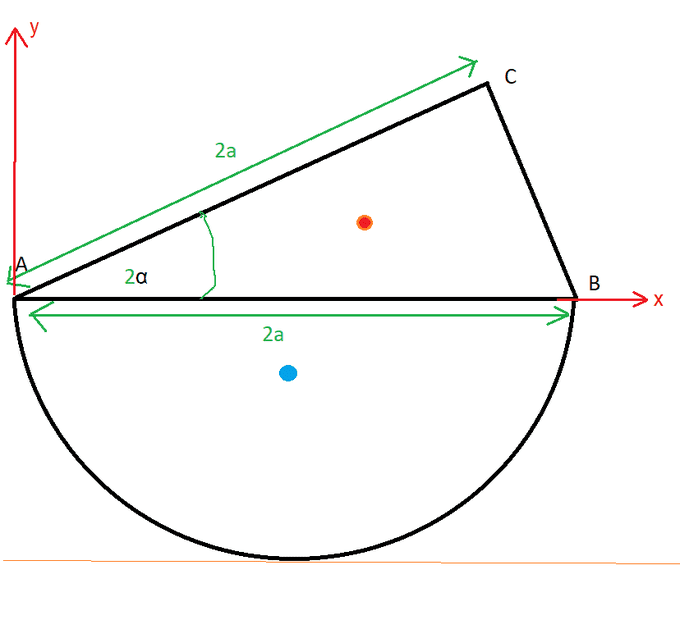The orange dot represents the centre of gravity of the triangle and the blue dot that of the hemisphere.

Let the weight of the hemisphere be W_h

So:

A is the point (0,0)
B is the point (2a,0)
C is the point ( 2a*cos(2ᾳ) , 2a*sin(2ᾳ) )

I can use the standard results for the centre of mass of a triangle and a hemisphere, so:

centre of mass of hemisphere is at (a,-3a/8)
centre of mass of triangle is at ( (2a + 2a*cos(2ᾳ)) / 3, 2a*sin(2ᾳ) / 3 )

If I say that the centre of gravity of the whole body is at (x_bar, y_bar) then taking moments about x_bar I can say:

W * ((2a + 2a*cos(2ᾳ)) / 3 - x_bar) = -W_h *(x_bar - a),

This would give me W_h in terms of a, W and ᾳ but also x_bar.

I could find x_bar by equating moments of the triangle, hemisphere and composite body about the y-axis, this starts to get complicated.

Can anyone help me see the way forward?

Thanks,
Mitch.

1) The question says AB=AC=2a which makes ΔABC isosceles: this is not what you have drawn.

2) In equilibrium the question says BC is vertical, which is not what you have drawn.

I would start by redrawing the diagram (with the x-axis horizontal). Note that the centre of gravity of the whole body will be vertically above the contact point of the hemisphere with the table.

gnits said:
A is the point (0,0)
B is the point (2a,0)
You realize that means your axes are not horizontal and vertical?

i drew the diagram with BC horizontal component of the center of mass of the whole system in the same vertical line as the point where it is rest upon on the table, I am getting the answer same as given with a cot(alpha) instead of cos(alpha)

•Delta2
bhupesh said:
i drew the diagram with BC horizontal component of the center of mass of the whole system in the same vertical line as the point where it is rest upon on the table, I am getting the answer same as given with a cot(alpha) instead of cos(alpha)
It is clearly not cos. As alpha tends to zero, AB tends to horizontal, so the hemisphere's mass needs to be arbitrarily large.

Thanks for the help so far. Firstly, sorry, my typo, it is cot not cos.
Here's a sketch with BC vertical.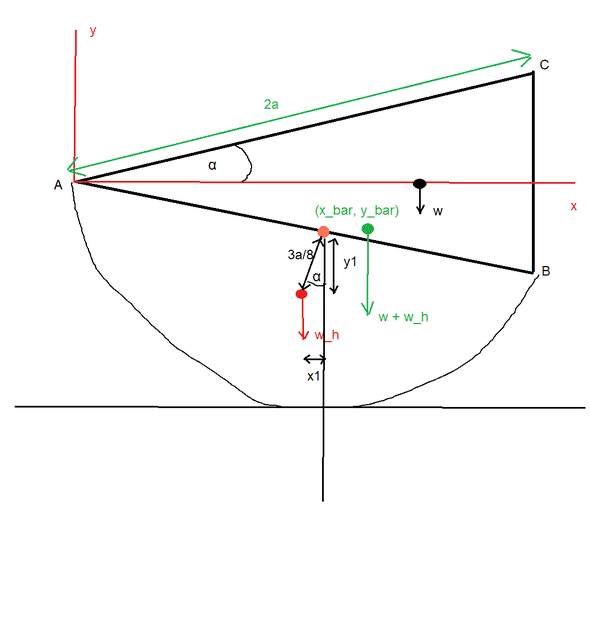Is the following the best way to go (sorry, preview not working for me so I am not using LaTeX in case I make errors):

Orange dot is midpoint of AB.

A(0,0), B(2a cos(α), -2a sin(α)), C(2a cos(α), 2a sin(α))

x1 = (3a/8)*sin(α)
y1 = (3a/8)*cos(α)
Midpoint of AB is (a cos(α), -a sin(α))
COG of ABC is (4a * cos(α) / 3, 0)
COG of hemisphere is ( a * cos(α) - (3a/8) * sin(α), -a sin(α) - (3a/8) * cos(α) )

Taking moments about (x_bar, y_bar) gives:

((4a/3) * cos(α) - x_bar) * w = -(x_bar - a cos(α) + (3a/8) * sin(α)) * w_h

So this would give w_h in terms of the required patameters if I knew x_bar in those terms.

Well, I could next equate the moment of the composite body about A with that of the sum of the moments of the triangle and hemisphere about A to get another equation for x_bar and them substitute this into the above eqaution. Am doing this but it is long winded, is this unnecessarily complicated, is there a better way? Thanks for any advice,

Thanks,
Mitch.

Last edited:
gnits said:
Here's a sketch with BC vertical.

View attachment 284149
Let 'P' be the point of contact of the hemisphere with the table.

In equilibrium, the overall CoG (x_bar, y_bar) will be vertically above P. This is not what your diagram shows.

As drawn, the system would be unstable rotate [minor edit made] clockwise. (Do you see why?)

Last edited:
Thanks for the help so far. Firstly, sorry, my typo, it is cot not cos.
Here's a sketch with BC vertical.

View attachment 284149

Is the following the best way to go (sorry, preview not working for me so I am not using LaTeX in case I make errors):

A(0,0), B(2a cos(α), -2a sin(α)), C(2a cos(α), 2a sin(α))

x1 = (3a/8)*sin(α)
y1 = (3a/8)*cos(α)
Midpoint of AB is (a cos(α), -a sin(α))
COG of ABC is (4a * cos(α) / 3, 0)
COG of hemisphere is ( a * cos(α) - (3a/8) * sin(α), -a sin(α) - (3a/8) * cos(α) )

Taking moments about (x_bar, y_bar) gives:

((4a/3) * cos(α) - x_bar) * w = -(x_bar - a cos(α) + (3a/8) * sin(α)) * w_h

So this would give w_h in terms of the required patameters if I knew x_bar in those terms.

Well, I could next equate the moment of the composite body about A with that of the sum of the moments of the triangle and hemisphere about A to get another equation for x_bar and them substitute this into the above eqaution. Am doing this but it is long winded, is this unnecessarily complicated, is there a better way? Thanks for any advice,

Thanks,
Mitch.
Steve4Physics said:
Let 'P' be the point of contact of the hemisphere with the table.

In equilibrium, the overall CoG (x_bar, y_bar) will be vertically above P. This is not what your diagram shows.

As drawn, the system would be unstable and tipple over clockwise. (Do you see why?)
Yes. I agree that the system as drawn would topple. My poor drawing. The curve of the hemisphere needs to be adjusted so that the contact point is below (x_bar, y_bar).

Here is a rough edit to hint at that: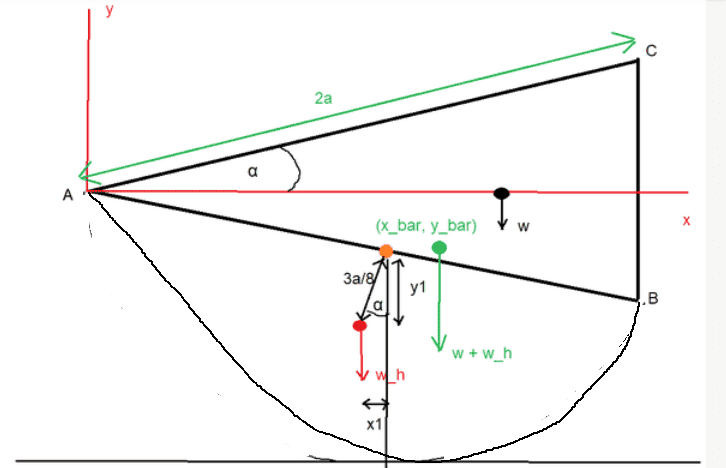Despite that unfortunate initial drawing, does my method seem the right way to go, i.e. equating moments about (x_bar, y_bar) and then about A?

gnits said:
Thanks for the help so far. Firstly, sorry, my typo, it is cot not cos.
Here's a sketch with BC vertical.

View attachment 284149

Is the following the best way to go (sorry, preview not working for me so I am not using LaTeX in case I make errors):

A(0,0), B(2a cos(α), -2a sin(α)), C(2a cos(α), 2a sin(α))

x1 = (3a/8)*sin(α)
y1 = (3a/8)*cos(α)
Midpoint of AB is (a cos(α), -a sin(α))
COG of ABC is (4a * cos(α) / 3, 0)
COG of hemisphere is ( a * cos(α) - (3a/8) * sin(α), -a sin(α) - (3a/8) * cos(α) )

Taking moments about (x_bar, y_bar) gives:

((4a/3) * cos(α) - x_bar) * w = -(x_bar - a cos(α) + (3a/8) * sin(α)) * w_h

So this would give w_h in terms of the required patameters if I knew x_bar in those terms.

Well, I could next equate the moment of the composite body about A with that of the sum of the moments of the triangle and hemisphere about A to get another equation for x_bar and them substitute this into the above eqaution. Am doing this but it is long winded, is this unnecessarily complicated, is there a better way? Thanks for any advice,

Thanks,
Mitch.

Yes. I agree that the system as drawn would topple. My poor drawing. The curve of the hemisphere needs to be adjusted so that the contact point is below (x_bar, y_bar).

Here is a rough edit to hint at that:

View attachment 284154
Despite that unfortunate initial drawing, does my method seem the right way to go, i.e. equating moments about (x_bar, y_bar) and then about A?
Your general approach is OK but I think that until you get the (misleading/incorrect) diagram sorted out you will not be able to solve this.

Point of contact with table is 'P. Centre of AB is 'Q'.

The overall CoG must be vertically above P (do you know why)? Note that PQ is a radius and the table's surface is a horizontal tangent to this radius.. This means that PQ is vertical. So the overall CoG lies on PQ - which is not what you have drawn.

With reference to Post #9, here's a diagram which should help.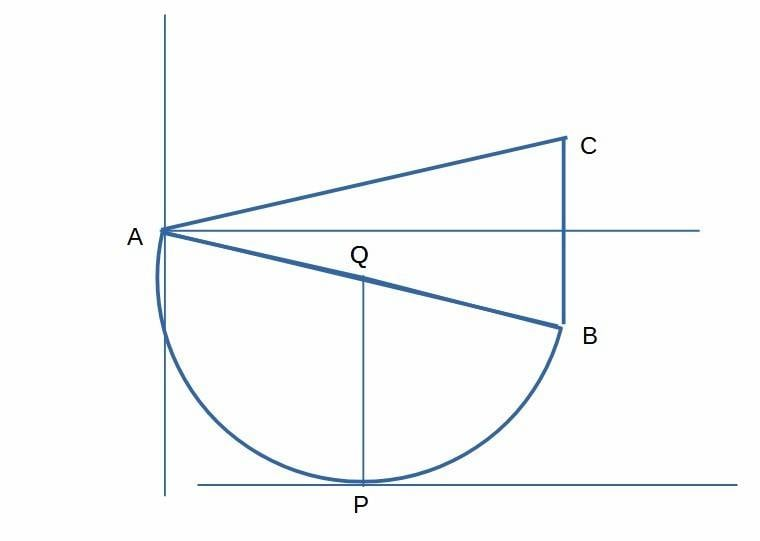The CoG lies on PQ.

Thanks very much everyone for your help. I see it now. My incorrect diagram sent me off on a much more complicated path. This is a very simple problem which was made into a tough one by my malformed diagram.

Lesson learned (hopefully!) thanks to all the great help,

Mitch.

•Steve4Physics
There is a second and final part to this question, and I am confused as to the final diagram, here is the additional question:

A particle of weight W is attached to a point P of AB where AP = (2/3)a and the body now settles in equilibrium with the midpoint of BC vertically above A. Prove that tan (α) = 1/2

So here is my diagram: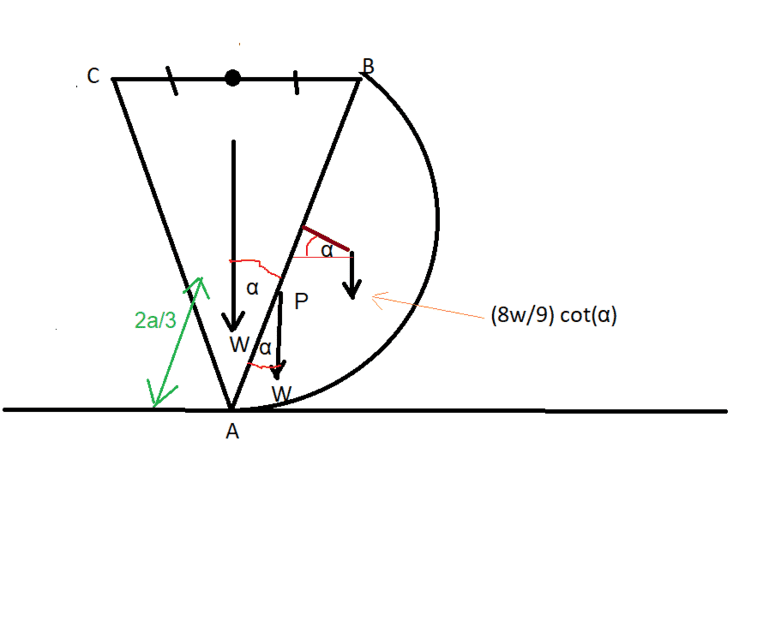Clearly I have misunderstood, as this could not balance on A as I have drawn it as all the weights are to the right of A.

Thanks for any help with the diagram,
Mitch.

gnits said:
There is a second and final part to this question, and I am confused as to the final diagram, here is the additional question:

A particle of weight W is attached to a point P of AB where AP = (2/3)a and the body now settles in equilibrium with the midpoint of BC vertically above A. Prove that tan (α) = 1/2

So here is my diagram:

View attachment 284166
Clearly I have misunderstood, as this could not balance on A as I have drawn it as all the weights are to the right of A.

Thanks for any help with the diagram,
Mitch.
It does not say A is touching the ground. It cannot be: the hemisphere will meet AB at a right angle.

haruspex said:
It does not say A is touching the ground. It cannot be: the hemisphere will meet AB at a right angle.
Thanks very much. Had trouble visualizing that correctly. I cut out a model to see it! Thanks very much for the help,

Mitch.